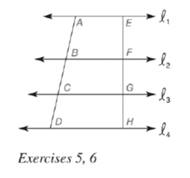Chapter 5.6, Problem 5EElementary Geometry For College St...

7th Edition
Alexander + 2 others
ISBN: 9781337614085

Solutions

Chapter
SectionElementary Geometry For College St...

7th Edition
Alexander + 2 others
ISBN: 9781337614085
Textbook Problem

Given: l 1 ∥ l 2 ∥ l 3 ∥ l 4 , A B = 5 , B C = 4 , C D = 3 , E H = 10 Find: E F , F G , G H (See the figure for Exercise 6.)To determine

To find:

EF,FG,GH.

Explanation

Given:

l1l2l3l4 and AB=5,BC=4,CD=3,EH=10.

Theorem used:

When three (or more) parallel lines are cut by a pair of transversals, the transversals are divided proportionally by the parallel lines.

If ab=cd then a+cb+d=ab=cd.

Calculation:

Given: l1l2l3l4

Since when three (or more) parallel lines are cut by a pair of transversals, the transversals are divided proportionally by the parallel lines.

ABEF=BCFG=CDGH

Also given that AB=5,BC=4,CD=3,EH=10.

Thus 5EF=4FG=3GH.

We know that, if ab=cd then a+cb+d=ab=cd.

5+4+3EF+FG+GH=5EF=4FG=3GH

From the figure, we see that EF+FG+GH=EH.

5+4+3EH=5EF=4FG=3GH

Given that EH=10

Still sussing out bartleby?

Check out a sample textbook solution.

See a sample solution

The Solution to Your Study Problems

Bartleby provides explanations to thousands of textbook problems written by our experts, many with advanced degrees!

Get Started

Fill in each blank: 3bu=pk

Elementary Technical Mathematics

The harmonic series is: 1 + 2 + 3 + 4 + …

Study Guide for Stewart's Multivariable Calculus, 8th

The center of 4x2 + 8x − 3y2 + 12y + 4 = 0 is (1, −2) (−1, 2) (2, −1) (−2, 1)

Study Guide for Stewart's Single Variable Calculus: Early Transcendentals, 8th